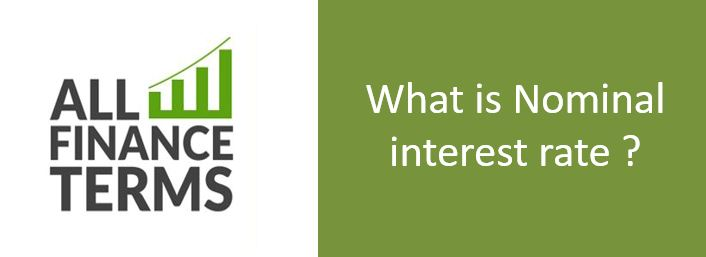## Definition of Nominal interest rate

Nominal interest rate is an interest rate which does not include any kind of inflation. It is the plain advertisement interest rate on loan. It does not include any compounding fee or interest.

### Brief Explanation of Nominal interest rate

For example, if a borrower borrows \$ 100 at an interest rate of 5 % then he is expected to pay \$ 5 without taking inflation into account.

Inflation and other costs are a confirm thing that is going to happen. To avoid inflation loss investors, prefer real interest rates. Hence real or effective interest rate exist in contrast to it.

For example if a borrower borrows \$ 100 from bank at the interest rate of 5 % an inflation rate is 2 % then the borrower is paying interest at 3 % and bank is receiving only 3 %. Hence bank’s purchasing power is increasing only by 3 %.

Inflation rate can never be estimated accurately by 100%.

Real interest rate = Nominal interest rate – inflation

Real interest rates can both be positive and negative.